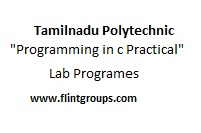# Tamilnadu Polytechnic : ” Programming in C “ Practical All Programs With sample Output UpdatedCourse Name : Diploma in Computer Engineering
Subject Code : 24036
Semester : III Semester
Subject Title : ” Programming in C “ Practical.

LAB EXERCISES

PART - A

2. Write a C Program to swap two variables using (i) third variable and (ii) without using a third variable.

3. Write a C Program to find the largest number between given three numbers.

4. Program to check whether the given string is palindrome or not.

6. Program to prepare the total marks for N students by reading the Regno,, Name, Mark1 to Mark6 by using array of structures. .

7. Write a function to calculate the sum and average of given three numbers. Write a main function to call the above function

8. Using pointers, find the length of the given string.

9. Write a program to print the address of a variable and increase the content by 5 and print the new value.

PART – B

2. Using Switch… Case Statement, print the given number into equivalent Word. ( For example if the input is 3, then the output should be THREE)

3. Write a program to find the factorial of a given number (i) Without recursion (ii) With recursion

4. Write a program to arrange the given N names in alphabetical order.

5. Write a program to read a string S1 from the terminal. Again read a string S2 from the terminal and check the given string S2 in the string S1. If it does, remove string S2 from the string S1 and print the updated string S1. ( For example S1 = Concatenate and S2 = cat , then the final result should be “Conenate”

6. Program to read ten values to an array variable. Use pointers to locate and display
each value.

7. Reverse the following using pointers ( i) String ( ii) N integer numbers stored in any
array.